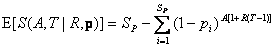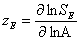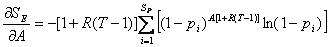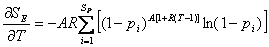Daniel J. McGlinn and Michael W. Palmer. 2009. Modeling the sampling effect in the species–time–area relationship. Ecology 90:836–846.

Appendix A. Derivation of the expected slope of the loglog speciesarea relationship, zE, and the expected slope of the loglog speciestime relationship, wE.

Proof:

From Eq. 3 in the main text the expected number of species is equal to:. (A.1)

The Arrhenius definition of z is the slope of the SAR in log-log space; therefore, we can define zE for the sampling model as the partial derivative of the natural logarithm (ln) of the expected richness as a function of the ln of area. For notational simplicity we will define this as:. (A.2)

Because Eq. A.1 is given with respect to area and not ln(area) and defined for SE and not ln(SE) we must use the chain rule to see that Eq. A.2 is actually:. (A.3)

And using the rules of differentiation for exponential functions and the chain rule once more we find that:. (A.4)

Combining this equation with Eq. A.3 we can see that the equation for zE is:. (A.5)

A similar process can be used to find wE.. (A.6)

And using the rules of differentiation for exponential functions and the chain rule we find that:. (A.7)

Combining this equation with Eq. A.6 we can see that the equation for wE is:. (A.8)

[Back to E090-057]